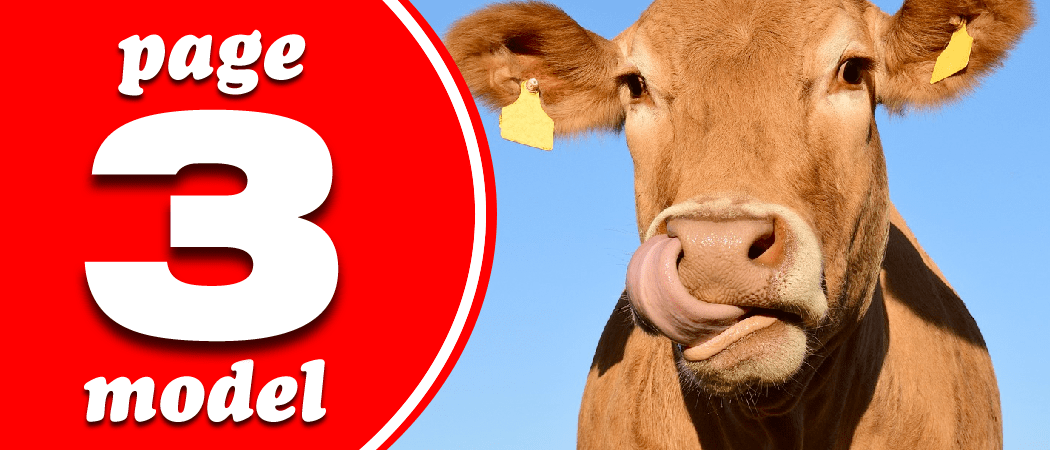# Page 3 model: Cows

How big is the biggest cow?Have you ‘herd’? The world’s largest cow is over six feet tall and weighs more than 1.3 tonnes. Is a bigger cow possi-bull? Will the future contain infinitely large cows? The steaks have never been higher!

To answer this question, let’s take a look at the cow’s legs. If the main (meaty) bit of the cow has a volume $V$ and density $\rho$ then its weight is $\rho V g$. So each leg supports a load of about
$N = \frac{\rho V g}{4}.$
In pursuit of glory, let’s now make the length, height and width of the cow bigger by a factor $a$. The cow’s new volume is $a^3 V$ and so the load on each leg is $a^3N$: it grows cubically as $a$ increases.

Can the legs cope? If we model the legs as cylinders (since they already ‘lactose’…), we can use a 1757 result from the famous cow enthusiast Euler: if a cylinder has height $L$ and radius $r$, the maximum load it can support standing upright is
$N_\text{max} = \frac{E \pi^3 r^4}{4 L^2}.$
$E$ here is just a property of the material: its stiffness, or Young’s moo-dulus.With our scaling, $L$ and $r$ are now $a$ times bigger. Our new maximum load is
$\frac{E\pi^3a^4r^4}{4a^2 L^2} = a^2 N_\text{max}.$

Uh oh… this only scales as $a^2$: quadratically.

So even though $N_\text{max}$ starts above $N$ (it has to, given that these cows exist!), there will come a maximum possible $a$, after which there will beef-ar too much cow and its legs will give way… an udder disaster.

This analysis tells us something really important about biology—that there is a natural maximum size for land mammals. But have we reached it for cows? Brody & Lardy’s 1000-page tome Bioenergetics and Growth from 1946 has all the de-tail you need. We’ll leave you to ruminate on the cow-culations.Adam is an assistant professor at Durham University, where he investigates weird, non-Newtonian fluids. If he’s not talking about the maths of chocolate fountains he is probably thinking about fonts, helping Professor Dirichlet answer your personal problems, and/or listening to BBC Radio 2.